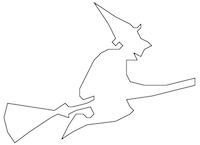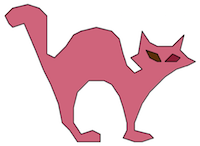# CS101 - Homework 9

Due: 2018-05-02 11:59p

#### Objectives

• Get some practice with Object-Oriented Programming
• Get some practice with files, and even more practice with lists

Let's make a connect-the-dots coloring book!

### [10 points] Prelab

Before coming to lab this week, complete the HW9 Prelab problem to draw a list of dots.

### [10 points] Draw the outline of a shape

In the Prelab, you created a Point class, and then a function that uses the turtle to draw dots given as a list of Point objects. We'll build on this basic functionality to create some connect-the-dots coloring shapes.

Write a function `drawLines(points)` that takes as input a list `points` of Point objects. Use the turtle to visit each point, thereby tracing the outline of the shape. I.e., connect the dots!

### [10 points] Reading in points from a file

We have provided a number of files that contain pairs of numbers that can be read as points. Each line contains an x-coordinate and a y-coordinate. See, for example, witch0.txt.

Write a function `readPoints(filename)` that opens the specified file, processes each line by creating a Point object with each pair of floating point numbers, and then returns a list of the Point objects.

### [10 points] Add functionality to scale points

In the prelab you created a Point class. Add a method `scale(factor)` to the Point class that modifies a Point by multiplying its x-coordinate and y-coordinate by a scale factor passed in as a parameter.

Write a function `scalePoints(points,factor)` that takes a list of Point objects and an integer `factor` as input parameters. For each point `p` in the list of Point objects, call `p.scale(factor)` to stretch out the points by `factor`.

### Putting it all together

Here we write a new function `drawObject(filename, stretch_factor)` that can draw a single shape such as this, defined in witch0.txt.You can use this code to put together all the components you've built thus far:
``````
def drawObject(filename, stretch_factor):
scalePoints(shape, stretch_factor)
t.tracer(False)
drawDots(shape)
t.tracer(True)
drawLines(shape)
t.hideturtle()
```
```

### [10 points] Extend to shapes with multiple parts

Once your program works with the file witch0.txt (which has just a single shape in it) let's continue to improve the program so it handles more complex point sets that allow breaks in the drawing.

Most files in hw09-files contain multiple points separated by the word "break". Between each occurrence of the word "break" is a point list for a component of the drawing that can be drawn with the pen down. But we'll need to pick up the pen between drawing these components.

One possible approach that minimizes the changes you need to make to your code: revise the `readPoints()` function so that it returns a list of lists. That is, between each occurrence of the word "break", we'll create a list of Point objects. When the word "break" is read, we'll end the current list of Point objects and add that sublist to the list of lists. Return the list of lists.

When you are done, you should be able to call your `drawObject()` function on all files in hw09-files such as cat.txt, witch.txt, etc. Make sure each data file is in the same folder as your python script.

### [2 extra points] Optional Challenge 1

Add color! You could include a color as an optional parameter to drawObject(). The witch and the cat are comprised of closed-in polygons that could be colored. For example:### [2 extra points] Optional Challenge 2

Keep tinkering... you could modify the Point class to allow a Point to be translated. Then figure out a way to draw multiple images on the same window.